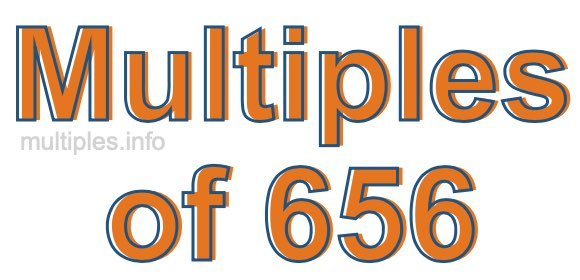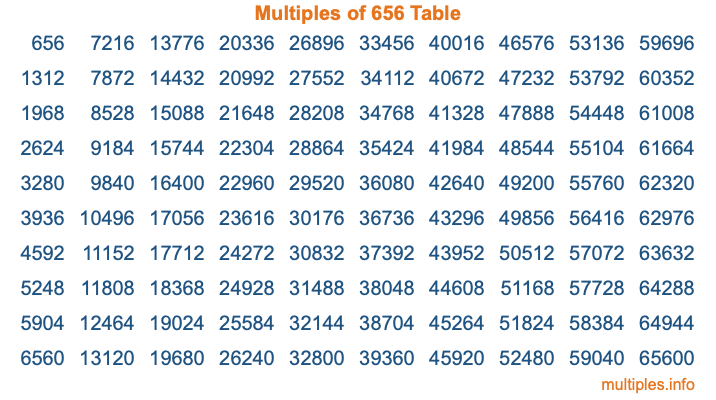Multiples of 656Welcome to the Multiples of 656 page. Here we will first teach you everything you will ever need to know about the multiples of 656, and then give you a study guide summary of everything we taught you to make sure you remember it all. Use this page to look up facts and learn information about the multiples of 656. This page will make you a multiples of six hundred fifty-six expert!

Definition of Multiples of 656
Multiples of 656 are all the numbers that when divided by 656 equal an integer. Each of the multiples of 656 are called a multiple. A multiple of 656 is created by multiplying 656 by an integer.

Therefore, to create a list of multiples of 656, you start with 1 multiplied by 656, then 2 multiplied by 656, then 3 multiplied by 656, and so on for as long as you want. Thus, the list of the first five multiples of 656 is 656, 1312, 1968, 2624, and 3280. To see a larger list of multiples of 656, see the printable image of Multiples of 656 further down on this page. We also have a category where you can choose any nth multiple of 656.

Multiples of 656 Checker
The Multiples of 656 Checker below checks to see if any number of your choice is a multiple of 656. In other words, it checks to see if there is any number (integer) that when multiplied by 656 will equal your number. To do that, we divide your number by 656. If the the quotient is an integer, then your number is a multiple of 656.

Is  a multiple of 656?

Least Common Multiple of 656 and ...
A Least Common Multiple (LCM) is the lowest multiple that two or more numbers have in common. This is also called the smallest common multiple or lowest common multiple and is useful to know when you are adding our subtracting fractions. Enter one or more numbers below (656 is already entered) to find the LCM.

Check out our LCM Calculator if you need more details about the Least Common Multiple or if you need the LCM for different numbers for adding and subtraction fractions.

nth Multiple of 656
As we stated above, 656 is the first multiple of 656, 1312 is the second multiple of 656, 1968 is the third multiple of 656, and so on. Enter a number below to find the nth multiple of 656.

th multiple of 656

Multiples of 656 vs Factors of 656
656 is a multiple of 656 and a factor of 656, but that is where the similarities end. All postive multiples of 656 are 656 or greater than 656. All positive factors of 656 are 656 or less than 656.

Below is the beginning list of multiples of 656 and the factors of 656 so you can compare:

Multiples of 656: 656, 1312, 1968, 2624, 3280, etc.

Factors of 656: 1, 2, 4, 8, 16, 41, 82, 164, 328, 656

As you can see, the multiples of 656 are all the numbers that you can divide by 656 to get a whole number. The factors of 656, on the other hand, are all the whole numbers that you can multiply by another whole number to get 656.

It's also interesting to note that if a number (x) is a factor of 656, then 656 will also be a multiple of that number (x).

Multiples of 656 vs Divisors of 656
The divisors of 656 are all the integers that 656 can be divided by evenly. Below is a list of the divisors of 656.

Divisors of 656: 1, 2, 4, 8, 16, 41, 82, 164, 328, 656

The interesting thing to note here is that if you take any multiple of 656 and divide it by a divisor of 656, you will see that the quotient is an integer.

Multiples of 656 Table
Below is an image of the first 100 multiples of 656 in a table. The table is in chronological order, column by column. The first column has the first ten multiples of 656, the second column has the next ten multiples of 656, and so on.The Multiples of 656 Table is also referred to as the 656 Times Table or Times Table of 656. You are welcome to print out our table for your studies.

Negative Multiples of 656
Although not often discussed or needed in math, it is worth mentioning that you can make a list of negative multiples of 656 by multiplying 656 by -1, then by -2, then by -3, and so on, to get the following list of negative multiples of 656:

-656, -1312, -1968, -2624, -3280, etc.

Multiples of 656 Summary
Below is a summary of important Multiples of 656 facts that we have discussed on this page. To retain the knowledge on this page, we recommend that you read through the summary and explain to yourself or a study partner why they hold true.

There are an infinite number of multiples of 656.

A multiple of 656 divided by 656 will equal a whole number.

656 divided by a factor of 656 equals a divisor of 656.

The nth multiple of 656 is n times 656.

The largest factor of 656 is equal to the first positive multiple of 656.

656 is a multiple of every factor of 656.

656 is a multiple of 656.

A multiple of 656 divided by a divisor of 656 equals an integer.

656 divided by a divisor of 656 equals a factor of 656.

Any integer times 656 will equal a multiple of 656.

Multiples of a Number
Here you can get the multiples of another number, all with the same attention to detail as we did for multiples of 656 on this page.

Multiples of
Multiples of 657
Did you find our page about multiples of six hundred fifty-six educational? Do you want more knowledge? Check out the multiples of the next number on our list!